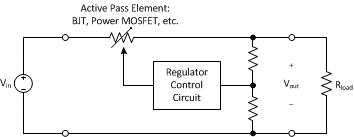# Tutorial: Linear Power Supplies

This tutorial installment is: Linear Power Supplies. This topic answers the following questions:

• What is a linear power supply?
• How do linear regulators control the output variable?
• What are the performance and physical characteristics of linear power supplies?

Last topic: Unregulated Power Supplies
Next topic: Efficiency

## Linear Power Supplies Introduction

In the last installment of the Power Supply Tutorial, we found that the most basic power supply was of a simple unregulated design. We also found that for all but the least demanding applications, the unregulated design is not able to maintain the output voltage close enough to the prescribed set point as the line voltage and load current changes. Thus, regulation methods have been developed to maintain the output voltage or current at a constant set point. The first type of regulated design was the linear regulator power supply.

The term “linear power supply” is typically thought of as a type of AC/DC system, providing a regulated output. The linear regulator is actually the part of the linear regulator power supply that performs the regulation.

## Linear Regulator Theory

Linear regulators employ a pass element serving as a variable resistor which forms a voltage divider with the load.The pass element functioning as a variable resistor can be semiconductor devices such as a bipolar junction transistor (BJT), power metal oxide semiconductor field effect transistor (MOSFET), insulated gate bipolar transistor (IGBT), or an electron tube such as a triode, tetrode, or pentode. Electron tubes would be used in highly specialized applications where there are no semiconductor devices suitable.

## Power Output Capability

A linear regulator can be designed to regulate power outputs as small as a watt or less. Linear regulators used alone in this fashion are performing DC/DC conversion. Linear power supplies can be designed to provide AC/DC conversion up to tens of kilowatts or even more. In this case the linear regulator is coupled with additional circuitry providing rectification and filtering.

## Noise and Ripple

Perhaps the most significant merit of linear power supplies is the cleanliness of the output voltage and the relative lack of electromagnetic emissions. The typical peak to peak output voltage ripple for a linear supply might be 1000x or 60dB less than the output DC level. So for a 5V output supply the typical peak to peak ripple voltage might be 5mV. A switching supply typically sees about a 100x or 40dB reduction. It is possible with careful design to achieve a 10,000x or 80dB reduction in a linear regulated power supply.

## Transient Response

The response of linear power supplies to line and load transients is better their switching counterparts because the linear supply does not have a switching frequency to limit the bandwidth. In linear supplies, the regulation bandwidth is typically limited by parasitic device elements.

## Weight and Size

The weight and size of the linear power supply is the major disadvantage. AC/DC conversion is done at low frequencies and therefore the transformer must be large to keep the core from saturating. This factor, along with efficiency to be discussed next, is the main reason why linear power supplies have limited use today. For example, a 500W linear power supply might weigh about 50 pounds and occupy a desktop. Whereas a 500W switching supply might weight less than 10 pounds and sit on a small part of the desktop.

## Efficiency

Linear regulators are typically thought of a being extremely inefficient – but that is not always the case and they can sometimes be more efficient that a switching power supply!

On a first order basis, the efficiency of a linear regulator is very simple to determine. The efficiency is simply the output voltage divided by the input voltage. If determined effort is made to keep the difference between the pass element input voltage and the output voltage as small as possible, the efficiency can be very good. This type of linear regulator is called a “low-dropout regulator”. For other cases where the operating point is not conducive to maintaining a low dropout, the linear regulator efficiency can suffer greatly.

As an example, if the input voltage in the figure above is 13.6 volts, and the regulator maintains an output voltage of 12 volts, for a voltage drop of 1.6 volts, the efficiency of the linear regulator is 12V/13.6V = 88.2%. The efficiency in this case is very good by most standards.

As another example, if the input voltage is 5 volts and the output voltage is 3.3 volts, for a voltage drop of 1.7 volts, the efficiency is 3.3V/5V = 66%. The efficiency in this case is not very good by most standards.

As a last example, if the input voltage is 5 volts and the output voltage is 1.8 volts, for a pass element voltage drop of 3.2 volts, the efficiency is 1.8V/5V = 36%. This efficiency in this case is very poor.

## Summary

In this article we found that linear power supplies excel in producing outputs with very low noise and ripple, low electromagnetic emissions, and have excellent transient response. However, they are by nature large and heavy when compared to switching power supplies. Linear power supplies would be the type of choice when outputs with low ripple and noise content are of paramount concern, and where larger size and substantially greater weight can be tolerated.

## Next Topic

The next tutorial installment is: Efficiency. This next topic will answer the following questions:

• What is power supply efficiency and how is it defined?
• How is power supply efficiency defined?
• What are the effects of efficiency on power converter design?

If you need assistance with power electronics design, call or email us today for help with your requirements. You can also go to our power electronics consultant website for more information about our services for business clients. Thank you for reading this tutorial article entitled “Linear Power Supplies”

Next topic: Efficiency
1.Raymond Koosha says: